Importance: Outstanding ✭✭✭✭
 Author(s): Berge, Claude Fulkerson, Delbert R.
 Subject: Graph Theory » Basic Graph Theory » » Matchings
 Keywords: cubic perfect matching
 Posted by: mdevos on: March 7th, 2007
Conjecture   Ifis a bridgeless cubic graph, then there exist 6 perfect matchingsofwith the property that every edge ofis contained in exactly two of.

This conjecture is due to Berge and Fulkerson, and appears first in [F] (see [S79b]).

Ifis 3-edge-colorable, then we may choose three perfect matchingsso that every edge is in exactly one. Taking each of these twice gives us 6 perfect matchings with the properties described above. Thus, the above conjecture holds trivially for 3-edge-colorable graphs. There do exist bridgeless cubic graphs which are not 3-edge-colorable (for instance the Petersen graph), but the above conjecture asserts that every such graph is close to being 3-edge-colorable.

Definition: An-graph is an-regular graphon an even number of vertices with the property that every edge-cut which separatesinto two sets of odd cardinality has size at least.

Observe that a cubic graph is a 3-graph if and only if it has no bridge. If G is an-regular graph which has an-edge-coloring, then every color class is a perfect matching, somust be even, and every color must appear in every edge-cut which separatesinto two sets of odd size, so every edge-cut of this form must have size at least. Thus, every-edge-colorable-regular graph is an-graph. In a sense, we may view the-graphs as the-regular graphs which have the obvious necessary conditions to be-edge-colorable. Seymour [S79b] defined-graphs and offered the following generalization of the Berge-Fulkerson conjecture.

Conjecture  (The generalized Berge-Fulkerson conjecture (Seymour))   Letbe an-graph. Then there existperfect matchings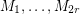ofwith the property that every edge ofis contained in exactly two of.

More generally, for a graph, one may consider the vector space of real numbers indexed by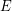. We associate every perfect matching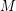with its characteristic vector. In this context, the Berge-Fulkerson conjecture asserts that for every 3-graph, the vector which is identically 1 may be written as a half-integer combination of perfect matchings. Edmonds matching polytope theorem [E] gives a complete characterization of what vectors in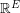which can be written as a nonnegative real combination of perfect matchings. A particular consequence of this theorem is that the vector which is identically 1 can be written as a nonnegative rational combination of perfect matchings if G is an-graph. It follows from this that for every-graph, there is a list of perfect matchings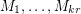so that every edge is contained in exactlyof them. Unfortunately, the particulardepends on the graph. The following weak version of the Berge-Fulkerson conjecture asserts that this dependence is inessential.

Conjecture  (the weak Berge-Fulkerson conjecture)   There exists a positive integerwith the following property. Every 3-graphhas a list ofperfect matchings such that every edge ofis contained in exactlyof them.

There is a fascinating theorem of Lovasz [L] that characterizes which vectors in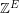can be written as an integer combination of perfect matchings. However, very little is known about nonnegative integer combinations of perfect matchings. In particular, if the Berge-Fulkerson conjecture is true, then for every 3-graph, there is a list of 5 perfect matchings with union(take any 5 of the 6 perfect matchings given by the conjecture). The following weakening of this (suggested by Berge) is still open.

Conjecture   There exists a fixed integersuch that the edge set of every 3-graph can be written as a union ofperfect matchings.

Another consequence of the Berge-Fulkerson conjecture would be that every 3-graph has 3 perfect matchings with empty intersection (take any 3 of the 6 perfect matchings given by the conjecture). The following weakening of this (also suggested by Berge) is still open.

Conjecture   There exists a fixed integersuch that every 3-graph has a list ofperfect matchings with empty intersection.

## Bibliography

[E] J. Edmonds, Maximum matching and a polyhedron with 0,1-vertices, J. Res. Nat. Bur Stand B, Math & Math Phys. 69B (1965), 125-130.

[F] D.R. Fulkerson, Blocking and anti-blocking pairs of polyhedra, Math. Programming 1 (1971) 168-194. MathSciNet

[KKN] T. Kaiser, D. Kral, and S. Norine, Unions of perfect matchings in cubic graphs

[L] L. Lovasz, Matching structure and the matching lattice, J. Combin. Theory Ser. B 43 (1987), 187-222. MathSciNet

[R] R. Rizzi, Indecomposable r-graphs and some other counterexamples, J. Graph Theory 32 (1999), 1-15. MathSciNet

[S79a] P.D. Seymour, "Some unsolved problems on one-factorizations of graphs", Graph theory and Related Topics, J.A. Bondy and U.S.R. Murty (Editors), Academic, New York (1979), 367-368.

[S79b] P.D. Seymour, On multi-colourings of cubic graphs, and conjectures of Fulkerson and Tutte, Proc. London Math Soc. 38 (1979), 423-460. MathSciNet

* indicates original appearance(s) of problem.